# MP Board Class 10th Maths Solutions Chapter 10 Circles Ex 10.2

In this article, we will share MP Board Class 10th Maths Book Solutions Chapter 10 Circles Ex 10.2 Pdf, These solutions are solved subject experts from the latest edition books.

## MP Board Class 10th Maths Solutions Chapter 10 Circles Ex 10.2

In questions 1 to 3, choose the correct option and give justification.

Question 1.
From a point Q, the length of the tangent to a circle is 24 cm and the distance of Q from the centre is 25 cm. The radius of the circle is
(A) 7 cm
(B) 12 cm
(C) 15 cm
(D) 24.5 cm
Solution:
(A): ∵ QT is a tangent to the circle at T and OT is radius∴ OT⊥QT
Also, OQ = 25 cm and QT = 24 cm
∴ Using Pythagoras theorem, we get
OQ2 = QT2 + OT2
⇒ OT2 = OQ2 – QT2 = 252 – 242 = 49
⇒ OT = 7
Thus, the required radius is 7 cm.

Question 2.
In figure, if TP and TQ are the two tangents to a circle with centre 0 so that ∠POQ =110°, then ∠PTQ is equal to
(A) 60°
(B) 70°
(C) 80°
(D) 90°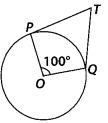Solution:
(B): TQ and TP are tangents to a circle with centre O and ∠POQ = 110°
∴ OP⊥PT and OQ⊥QT
⇒ ∠OPT = 90° and ∠OQT = 90°
Now, in the quadrilateral TPOQ, we get
∠PTQ + 90° + 110° + 90° = 360° [Angle sum property of a quadrilateral]
⇒ ∠PTQ + 290° = 360°
⇒ ∠PTQ = 360° – 290° = 70°Question 3.
If tangents PA and PB from a point P to a circle with centre O are inclined to each other at angle of 80°, then ∠POA is equal to
(A) 50°
(B) 60°
(C) 70°
(D) 80°
Solution:
(A) : Since, O is the centre of the circle and two tangents from P to the circle are PA and PB.
∴ OA⊥AP and OB⊥BP
⇒ ∠OAP = ∠OBP = 90°Now, in quadrilateral PAOB, we have
∠BPA + ∠PAO + ∠AOB + ∠OBP = 360°
⇒ 80° + 90° + ∠AOB + 90° = 360°
⇒ 260° + ∠AOB = 360°
⇒ ∠AOB = 360° – 260° ⇒ ∠AOB = 100°
In right ∆OAP and right ∆OBP, we have
OP = OP [Common]
∠OAP = ∠OBP [Each 90°]
OA = OB [Radii of the same circle]
∴ ∆OAP ≅ ∆OBP [By RHS congruency]
⇒ ∠POA = ∠POB [By CPCT]
∴ ∠POA = $$\frac{1}{2}$$ ∠AOB = $$\frac{1}{2}$$ × 100° = 50°

Question 4.
Prove that the tangents drawn at the ends of a diameter of a circle are parallel.
Solution:
In the figure, PQ is diameter of the given circle and O is its centre.
Let tangents AB and CD be drawn at the end points of the diameter PQ.
Since, the tangents at a point to a circle is perpendicular to the radius through the point.∴ PQ⊥AB
⇒ ∠APQ = 90°
And PQ⊥CD
⇒ ∠PQD = 90° ⇒ ∠APQ = ∠PQD
But they form a pair of alternate angles.
∴ AB || CD
Hence, the two tangents are parallel.

Question 5.
Prove that the perpendicular at the point of contact to the tangent to a circle passes through the centre.
Solution:
In the figure, the centre of the circle is O and tangent AB touches the circle at P. If possible, let PQ be perpendicular to AB such that it is not passing through O.
Join OP.
Since, tangent at a point to a circle is perpendicular to the radius through that point.∴ OP⊥AB
⇒ ∠OPB = 90° ……….. (1)
But by construction, PQ⊥AB
⇒ ∠QPB = 90° ………….. (2)
From (1) and (2),
∠QPB = ∠OPB
which is possible only when O and Q coincide. Thus, the perpendicular at the point of contact to the tangent to a circle passes through the centre.

Question 6.
The length of a tangent from a point A at distance 5 cm from the centre of the circle is 4 cm. Find the radius of the circle.
Solution:
∵ The tangent to a circle is perpendicular to the radius through the point of contact.
∴ ∠OTA = 90°
Now, in the right ∆OTA, we have
OA2 = OT2 + AT2 [Pythagoras theorem]⇒ OT2 = 52 – 42
⇒ OT2 = (5 – 4)(5 + 4)
⇒ OT2 = 1 × 9 = 9 = 32
⇒ OT = 3
Thus, the radius of the circle is 3 cm.Question 7.
Two concentric circles are of radii 5 cm and 3 cm. Find the length of the chord of the larger circle which touches the smaller circle.
Solution:
In the figure, O is the common centre, of the given concentric circles.
AB is a chord of the bigger circle such that it is a tangent to the smaller circle at P.
Since, OP is the radius of the smaller circle.
∴ OP⊥AB ⇒ ∠APO = 90°
Also, radius perpendicular to a chord bisects the chord.∴ OP bisects AB
⇒ AP = $$\frac{1}{2}$$ AB
Now, in right ∆APO,
OA2 = AP2 + OP2
⇒ 52 = AP2 + 32 ⇒ AP2 = 52 – 32
⇒ AP2 = 42 ⇒ AP = 4 cm
⇒ $$\frac{1}{2}$$ AB = 4 ⇒ AB = 2 × 4 = 8 cm
Hence, the required length of the chord AB is 8 cm.

Question 8.
A quadrilateral ABCD is drawn to circumscribe a circle (see figure).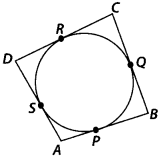Prove that AB + CD = AD + BC
Solution:
Since, the sides of quadrilateral ABCD, i.e., AB, BC, CD and DA touches the circle at P, Q, R and S respectively, and the lengths of two tangents to a circle from an external point are equal.
∴ AP = AS, BP = BQ,
DR = DS and CR = CQ
(AP + BP) + (CR + RD) = (BQ + QQ) + (DS + SA)
⇒ AB + CD = BC + DA

Question 9.
In figure, XY and X’Y’ are two parallel tangents to a circle with centre O and another tangent AB with point of contact C intersecting XY at A and X’Y’ at B.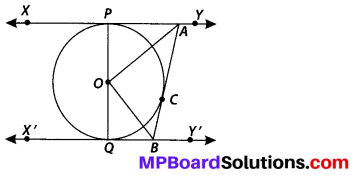Prove that ∠AOB = 90°.
Solution:
∵ The tangents drawn to a circle from an external point are equal.
∴ AP = AC ……… (1)
Join OC.
In ∆PAO and ∆CAO, we have
AO = AO [Common]
OP = OC [Radii of the same circle]
AP = AC [From (1)]
⇒ ∆PAO ≅ ∆CAO [SSS congruency]
∴ ∠PAO = ∠CAO
⇒ ∠PAC = 2∠CAO …………. (2)
Similarly, ∠CBQ = 2∠CBO ……………… (3)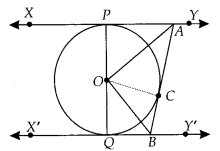Again, we know that sum of internal angles on the same side of a transversal is 180°.
∴ ∠PAC + ∠CBQ = 180°
2∠CAO + 2∠CBO = 180° [From (2) and (3)]
⇒ ∠CAO + ∠CBO = $$\frac{180^{\circ}}{2}$$ = 90° …………… (4)
Also, in ∆AOB,
∠BAO + ∠OBA + ∠AOB = 180° [Sum of angles of a triangle]
⇒ ∠CAO + ∠CBO + ∠AOB = 180°
⇒ 90° + ∠AOB = 180° [From (4)]
⇒ ∠AOB = 180° – 90°
⇒ ∠AOB = 90°

Question 10.
Prove that the angle between the two tangents drawn from an external point to a circle is supplementary to the angle subtended by the line-segment joining the points of contact at the centre.
Solution:
Let PA and PB be two tangents drawn from an external point P to a circle with centre O.Now, in right ∆OAP and right ∆OBP, we have
PA = PB [Tangents to circle from an external point]
OA = OB [Radii of the same circle]
OP = OP [Common]
⇒ ∆OAP ≅ ∆OBP [By SSS congruency]
∴ ∠OPA = ∠OPB [By CPCT]
and ∠AOP = ∠BOP
⇒ ∠APB = 2∠OPA and ∠AOB = 2∠AOP
In right ∆OAP,
∠AOP + ∠OPA + ∠PAO = 180°
⇒ ∠AOP = 180° – 90° – ∠OPA
⇒ ∠AOP = 90° – ∠OPA
⇒ 2∠AOP = 180° – 2∠OPA
⇒ ∠AOB = 180° – ∠APB
⇒ ∠AOB + ∠APB = 180°Question 11.
Prove that the parallelogram circumscribing a circle is a rhombus.
Solution:
We have ABCD, a parallelogram which circumscribes a circle (i.e., its sides touch the circle) with centre O.
Since, tangents to a circle from an external point are equal in length∴ AP = AS
BP = BQ
CR = CQ
DR = DS
(AP + BP) + (CR + DR) = (AS + DS) + (BQ + CQ)
⇒ AB + CD = AD + BC
But AB = CD [Opposite sides of parallelogram]
∴ AB + CD = AD + BC ⇒ 2AB = 2BC
⇒ AB = BC
Similarly, AB = DA and DA = CD
Thus, AB = BC = CD = DA
Hence, ABCD is a rhombus.

Question 12.
A triangle ABC is drawn to circumscribe a circle of radius 4 cm such that the segments BD and DC into which BC is divided by the point of contact D are of lengths 8 cm and 6 cm respectively (see figure). Find the sides AB and AC.Solution:
Here ∆ABC circumscribes the circle with centre O. Also, radius = 4 cm
Let AC and AB touches the circle at E and F, respectively and join OE and OF.
∵ The sides BC, CA and AB touches the circle at D, E and F respectively.
∴ BF = BD = 8 cm
[ ∵ Tangents to a circle from an external point are equal]
CD = CE = 6 cm
AF = AE = x cm (say)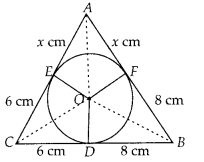∴ The sides of the ∆ABC are 14 cm, (x + 6) cm and (x + 8) cm
Perimeter of ∆ABC
= [14 + (x + 6) + (x + 8)] cm
= [14 + 6 + 8 + 2x] cm
= (28 + 2x) cm
⇒ Semi perimeter of ∆ABC,
s = $$\frac{1}{2}$$ [28 + 2x] cm = (14 + x) cm
∴ s – a = (14 + x) – (8 + x) = 6
s – b = (14 + x) – (14) = x
s – c = (14 + x) – (6 + x) = 8
where, a = AB, b = BC, c = ACSquaring both sides, we get
(14 + x)2 = (14 + x)3x
⇒ 196 + x2 + 28x = 42x + 3x2
⇒ 2x2 + 14x – 196 = 0
⇒ x2 + 7x – 98 = 0
⇒ (x – 7)(x + 14) = 0
⇒ x – 7 = 0 or x + 14 = 0
⇒ x = 7 or x = -14
But x = -14 is rejected.
∴ x = 7
Thus, AB = 8 + 7 = 15 cm, BC = 8 + 6 = 14 cm and CA = 6 + 7 = 13 cm

Question 13.
Prove that opposite sides of a quadrilateral circumscribing a circle subtend supplementary angles at the centre of the circle.
Solution:
We have a circle with centre O. A quadrilateral ABCD is such that the sides AB, BC, CD and DA touches the circle at P, Q, R and S respectively.
Join OP, OQ, OR and OS.
We know that two tangents drawn from an external point to a circle subtend equal angles at the centre.∴ ∠1 = ∠2
∠3 = ∠4
∠5 = ∠6 and ∠7 = ∠8
Also, the sum of all the angles around a point is 360°.
∴ ∠1 + ∠2 + ∠3 + ∠4 + ∠5 + ∠6 + ∠7 + ∠8 = 360°
∴ 2(∠1 + ∠8 + ∠5 + ∠4) = 360°
⇒ (∠1 + ∠8 + ∠5 + ∠4) = 180° …………. (1)
and 2(∠2 + ∠3 + ∠6 + ∠7) = 360°
⇒ (∠2 + ∠3 + ∠6 + ∠7) = 180° ……………. (2)
Since, ∠2 + ∠3 = ∠AOB, ∠6 + ∠7 = ∠COD, ∠1 + ∠8 = ∠AOD and ∠4 + ∠5 = ∠BOC
∴ From (1) and (2), we have
∠AOD + ∠BOC = 180°
and ∠AOB + ∠COD = 180°